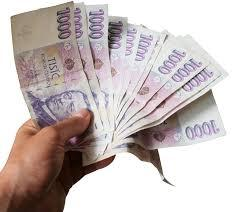# Increased 4898

The price of flour increased three times in a row by 10% each time. The shop then reduced it by 30%. Now the price of flour is 5.60 CZK. What was the original price?

x =  6.0105 Kc

### Step-by-step explanation:

x · (1+10/100)·(1+10/100)·(1+10/100)·(1-30/100) = 5.60

93.17x = 560

x = 560/93.17 = 6.01051841

x = 6.010518

Our simple equation calculator calculates it.Did you find an error or inaccuracy? Feel free to write us. Thank you!

Tips for related online calculators